Next: Time-Frequency-Scale Volume Up: THE CHIRPLET Previous: Gaussian Chirplet

## Notation

The family of chirplets is generated from the mother chirplet by applying simple parameterized mathematical operations to it. The parameters of these operations form an index into the chirplet family.

The operations corresponding to the coordinate axes of the chirplet transform parameter space are presented in Table 1. The operators will be explained as they are used. The general notion to keep in mind is that any combination of these operators results in a 2-D affine coordinate transformation in the TF plane, which may be represented using the homogeneous coordinates often used in computer graphicsfoley.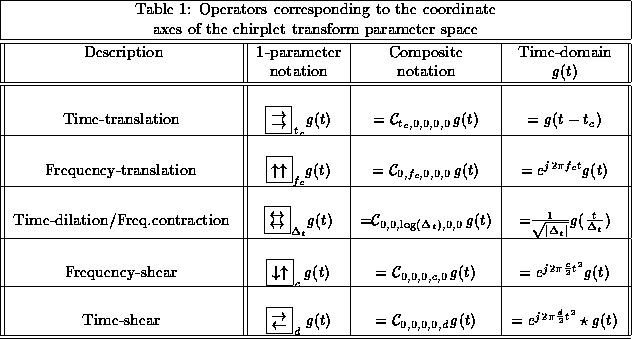The continuous STFT may be formulated as an inner product of the signal with the family of functions given in (2):

S_t_c,f_c = g_t_c,f_c,(_t) | s(t)

where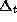is a suitably-chosen (fixed) window size, and s(t) is the original signal. We use the Dirac inner product notation, defined by:

g | s = _-^ \; g^*(t) s(t) \; dt

where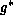denotes the complex conjugate of g. We use the vertical bar between the arguments and absorb the conjugation into the first element so that we can write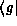by itself, as an operator that acts on whatever follows, in this case the signal,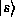.

Suppose we take the Gaussian window, centered at t=0, with unit pulse duration, as given by:

g(t) = 1 (-12 t^2)

We denote a time shift to the position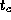, with an operator that has a multiplicative law of composition: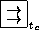(Table 1). A frequency shift to the position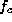consists of multiplying the window by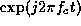, which we will denote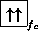. The single-operator notation (Table 1, second column) consists of a pictorial icon depicting the effect each operator has on the TF plane, even when the operator is acting in the time-domain. For example the symbol with the two up-arrows indicates a uniform upward shift along the frequency axis, of the time-frequency plane, for positive values of the parameter. These pictorial icons are consistent with our observation that each of these operators acts in the time domain to perform an area-preserving affinecoordinate transformation in the time-frequency plane.

Using the new notation, we can re-write (4) as:

S_t_c,f_c = _\: t_c _f_c g \: \: s

where we have also eliminated the time coordinate, recognizing that for any operator in the time-domain, there is an equivalent operator in the frequency domain, or in the TF plane, or in whatever other reasonable coordinate space one might wish to work in. The multiplicative law of composition of the operators is applied in the order that they appear from right to left (e.g.is applied first, and then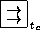is applied to that result). Note that these two operators do not commute. Adopting the convention of applying the frequency-shift first, and then the time-shift, results in the term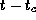appearing in the second exponent of (2). Applying the operators in the reverse order would result in a different phase-shift. In order to form a true group, we need a third parameter,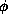, to indicate the degree to which the two operators do not commute. Such a group structure is known as the Heisenberg groupfolland. If we are only interested in the magnitude of the TF plane (e.g. the spectrogram) then we can simply consider the two-dimensional (two-parameter) translational group, and describe the operations in terms of this simpler group. Both (4) and (7) are equivalent, providing us with some measure of the signal energy around coordinates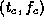, but (7) emphasizes the fact that the STFT is a correlation between members of a two-parameter family of time-shifted and frequency-shifted versions of the same primitive, g.

Using the simplified law of composition, we may compose a time shift bywith a frequency shift by, as follows:

_\: t_c _f_c = C_t_c,0,0,0,0 C_0,f_c,0,0,0 = C_t_c,f_c

where omissions from the parameter list of C indicate values of zero.

Equation 7) may be re-written, using the ``Composite notation'' (Table 1, third column):

S_t_c,f_c = C_t_c,f_c g(t) \: \: s(t)Next: Time-Frequency-Scale Volume Up: THE CHIRPLET Previous: Gaussian Chirplet

Steve Mann
Thu Jan 8 19:50:27 EST 1998